# SSC CGL EXAMS 2018 | Quantitative Aptitude Practice Questions (Day-10)

Dear Aspirants, Here we have given the Important SSC Exam 2018 Practice Test Papers. Candidates those who are preparing for SSC 2018 can practice these questions to get more confidence to Crack SSC 2018 Examination.

[WpProQuiz 4233]

1)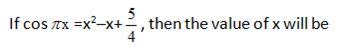a) 1

b) 2

c) 3

d) None of these

2) Find the minimum value of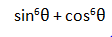a) ¾

b) ¼

c) 1

d) ∞

3) The angles of elevation of the tap of a tower from two points situated at distance 36 m and 64m from its base and in the same straight line with it are complementary. What is the height of the tower?

a) 50 m

b) 48 m

c) 25 m

d) 24m

5) Sonu can do a piece of work is 120 days and Dinesh can finish it 150 days. They together worked for 10 days. After that Sonu leave the tab. Thereafter Ramesh joins and the rest work is completed by Ramesh where efficiency of Ramesh   according to the statement that Ramesh completed the given work in 60 days. In how many days total work will be completed?

a) 61

b) 71

c) 80

d) 06

5) On a sum of money, the simple interest for 2 years is 480, white the compound interest is Rs. 508.80, the rate of interest being the some in both the cases. The rate of interest is—

a) 10%

b) 15%

c) 12%

d) None of these

6) If a2 + 4b2 = 4ab then a : b = ?

a) 1 : 2

b) 2 : 1

c) 4 : 1

d) 1 : 4

7) At the beginning of a partnership business the capital of Binod was  times more than that of Amar. After 6 months, Binod withdrew ½ of his capital and after 10 months Amar withdrew ¼ of his capital. At the end of the year, if the profit incurred is Rs. 106,000, find the amount received by Amar?

a) 40000

b) 42000

c) 44000

d) 46000

8) A travel agent sold a travel ticket for Rs 3,450 and make a profit of 20% in the transaction find his loss percent if he had sold his items for Rs. 2000.

a) 30.43%

b) 40.03%

c) 40%

d) None of These

9) In  ABC, O is the centre d and AD, BE and CF are three medians and the area of AOE = 30 cm2. The area of the quadrilateral BDOF is.

a) 60 cm2

b) 40 cm2

c) 15cm2

d) 50 cm2

10) In what ratio, the line made by joining the points A (—4, —3) and B (5, 2) intersects X-axis?

a) 3 : 2

b) 2 : 3

c) —3 : 2

d) — 2 : 3

11) From a point A on the ground, the angle of elevation of the top of a 20 m tall building is 45o. A flag is hoisted at the top of the building and the angle of elevation of the top of the flagstaff from A is 60o. Find the length of flagstaff.

a) 15.23m

b) 14.64m

c) 14m

d) 15.65m

12) Three sets of English, Mathematics and Science books containing 336, 240 and 96 books, respectively have to stack in such a way that all the books are stored subject wise and height of each stack is same. Total number of stacks will be

a) 14

b) 22

c) 21

d) 48

13) A moving train, 66 m overtakes another train of 88m long, moving in the same direction in 0.168 min. If the second train is moving at 30 km/h, at what speed is the first train moving?

a) 85 km/h

b) 55 km/h

c) 50 km/h

d) 25 km/h

14) If the positions of the digits of a two-digit number are interchanged, the number obtained is smaller than the original number by 27. If the digits of the number are in the ratio of 1 : 2, then what is the original number?

a) 36

b) 48

c) 63

d) 56

15) Numbers a1, a2, a3, a4, a5, …………… a24 with common difference= 10, are in arithmetic progression and

a1 + a5 + a10+a15 + a20 + a25 = 225.

The value of

a1 + a2 + a3 + a4 + a5 + ……+ a23 + a24is

a) 525

b) 725

c) 860

d) 900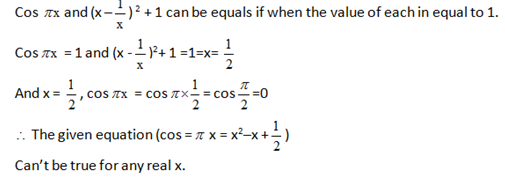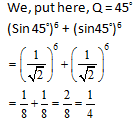By short trick:

AB2 = BD X BC

h2 = 36 X 64

h = 6 X 8 = 48 m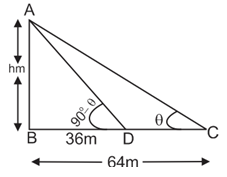According to the questions:

L C M = 600

Sonu = 120 days = 5 Units

Dinesh = 150 days = 4 Units

S + D = 9 Units

Work of 10 days by Sonu and Dinesh (5+4) * 10 = 90 work, Remaining work

= 600 – 90 = 510 work

Remaining work is done by Ramesh = 510/ 10 = 51 days

Total Day = 51 + 10 = 61 days.

Rs. 480 S.I for 2 years

∴ Rs. 240 S.I for 1 years.

And Rs. 508.80 is C.I for 2 years

In 1st year S.I and C.I in same hence 2nd year only C.I is Rs 4 08.80 – Rs 480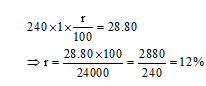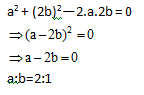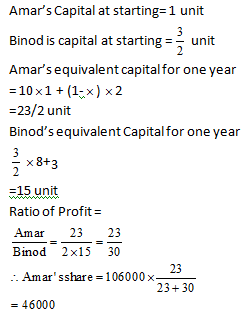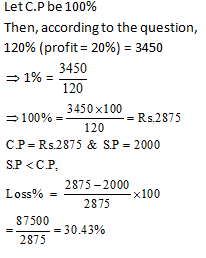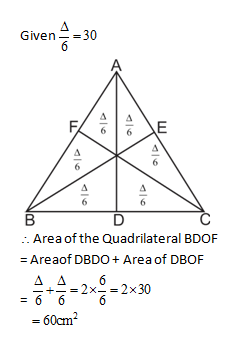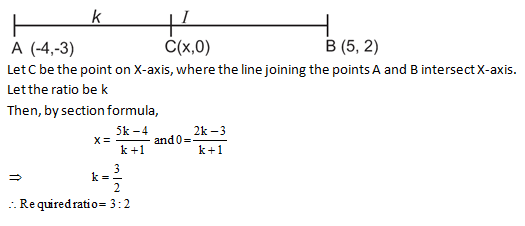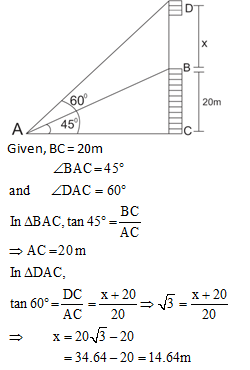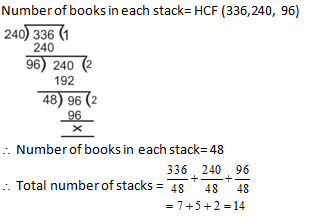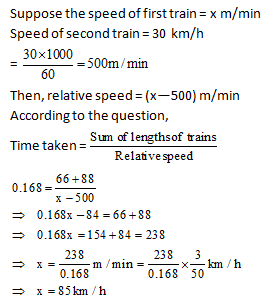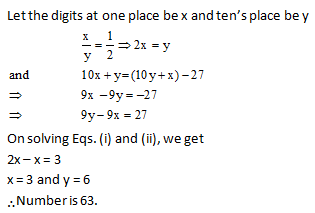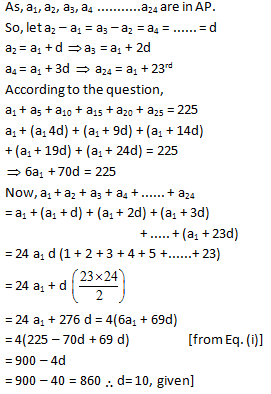### *******************